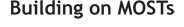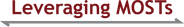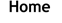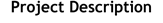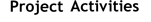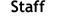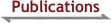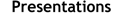Learning to Become a Researcher in an Ongoing Research Project: A Communities of Practice Perspective

Arslan, O., Van Zoest, L. R., & Ruk, J. M. (2017). Learning to become a researcher in an ongoing research project: A communities of practice perspective. In E. Galindo, & J. Newton (Eds.), Proceedings of the 39th annual meeting of the North American Chapter of the International Group for the Psychology of Mathematics Education (pp. 1423–1426). Indianapolis, IN: Hoosier Association of Mathematics Teacher Educators.

We apply Wenger's (1998) communities of practice ideas to the process of incorporating new researchers into an ongoing mathematics education research project. We illustrate this application by describing how the Leveraging MOSTs research project coding team can be viewed as a community of practice. We describe how we have used this particular community of practice to bring new researchers into the project, and new researchers reflect on their experiences with the coding team. Mutual engagement in project work with experienced researchers and having a rich shared repertoire to draw on led to the new researchers developing a shared understanding of the project and being successfully incorporated into the MOST community. This work speaks to the importance of deliberately creating communities of practice for new mathematics researchers to participate within.

Teachers' Responses To a Common Set of High Potential Instances of Student Mathematical Thinking

Stockero, S. L., Van Zoest, L. R., Peterson, B. E., Leatham, K. R., & Rougée, A. O. T. (2017). Teachers' responses to a common set of high potential instances of student mathematical thinking. In E. Galindo, & J. Newton (Eds.), Proceedings of the 39th annual meeting of the North American Chapter of the International Group for the Psychology of Mathematics Education (pp. 1178–1185). Indianapolis, IN: Hoosier Association of Mathematics Teacher Educators.

This study investigates teacher responses to a common set of high potential instances of student mathematical thinking to better understand the role of the teacher in shaping meaningful mathematical discourse in their classrooms. Teacher responses were coded using a scheme that disentangles the teacher move from other aspects of the teacher response, including who the response is directed to and the degree to which the student thinking is honored. Teachers tended to direct their response to the student who had shared their thinking and to explicitly incorporate ideas core to the student thinking in their response. We consider the nature of these responses in relation to principles of productive use of student mathematical thinking.

Beyond the "Move": A Scheme for Coding Teachers' Responses To Student Mathematical Thinking

Peterson, B. E., Van Zoest, L. R., Rougée, A. O. T., Freeburn, B., Stockero, S. L., & Leatham, K. R. (2017). Beyond the "move": A scheme for coding teachers' responses to student mathematical thinking. In B. Kaur, W. K. Ho, T. L. Toh, & B. H. Choy. (Eds.), Proceedings of the 41st annual meeting of the International Group for the Psychology of Mathematics Education, Vol. 4 (pp. 17–24). Singapore: International Group for the Psychology of Mathematics Education.

To contribute to the field's understanding of the teachers' role in using student thinking to shape classroom mathematical discourse, we developed the Teacher Response Coding Scheme (TRC). The TRC provides a means to analyze teachers' in-the-moment responses to student thinking during instruction. The TRC differs from existing schemes in that it disentangles the teacher move from the actor (the person publically asked to consider the student thinking), the recognition (the extent to which the student recognizes their idea in the teacher move), and the mathematics (the alignment of the mathematics in the teacher move to the mathematics in the student thinking). This disentanglement makes the TRC less value-laden and more useful across a broad range of settings.

Attributes of Instances of Student Mathematical Thinking That Are Worth Building on in Whole-Class Discussion

Van Zoest, L. R., Stockero, S. L., Leatham, K. R., Peterson, B. E., Atanga, N. A., & Ochieng, M. A. (2017). Attributes of instances of student mathematical thinking that are worth building on in whole-class discussion. Mathematical Thinking and Learning, 19, 33–54.

This study investigated attributes of 278 instances of student mathematical thinking during whole-class interactions that were identified as having high potential, if made the object of discussion, to foster learners' understanding of important mathematical ideas. Attributes included the form of the thinking (e.g., question vs. declarative statement), whether the thinking was based on earlier work or generated in the moment, the accuracy of the thinking, and the type of thinking (e.g., sense-making). Findings illuminate the complexity of identifying student thinking worth building on during whole-class discussion and provide insight into important attributes of these high potential instances that could be used to help teachers more easily recognize them. Implications for researching, learning, and enacting the teaching practice of building on student mathematical thinking are discussed.

Noticing Distinctions Among and Within Instances of Student Mathematical Thinking

Stockero L. S., Leatham, K. R., Van Zoest, L. R., & Peterson, B. E. (2017) Noticing Distinctions Among and Within Instances of Student Mathematical Thinking. In E. Schack, J. Wilhelm, & M. H. Fisher (Eds.), Teacher noticing: A hidden skill of teaching (pp. 467). Cham, Switzerland: Springer International.

In this chapter, we argue that there are two critical aspects of noticing student mathematical thinking: noticing within an instance of student thinking and noticing among instances of student thinking. We use the noticing literature to illustrate these distinctions. We then discuss how the MOST Analytic Framework analysis provides structure and guidance for noticing both within and among instances, and illustrate the complex interaction of these two types of noticing through the analysis of an excerpt of classroom dialogue. We conclude by offering the perspective that studies of noticing must go beyond placing value on student mathematical thinking to discriminating among instances of student thinking based on their potential to be used to support students' understanding of important mathematics.

Imprecision in Classroom Mathematics Discourse

Leatham, K. R., Peterson, B. E., Merrill, L., Van Zoest, L. R., & Stockero, S. L. (2016). Imprecision in classroom mathematics discourse. In M. B. Wood, E. E. Turner, M. Civil, & J. A. Eli (Eds.), Proceedings of the 38th Annual Meeting of the North American Chapter of the International Group for the Psychology of Mathematics Education (pp. 1236–1243). Tucson, AZ: University of Arizona.

We theorize about ambiguity in mathematical communication and define a certain subset of ambiguous language usage as imprecise. For us, imprecision in classroom mathematics discourse hinders in-the-moment communication because the instance of imprecision is likely to create inconsistent interpretations of the same statement among individuals. We argue for the importance of attending to such imprecision as a critical aspect of attending to precision. We illustrate various types of imprecision that occur in mathematics classrooms and the ramifications of not addressing this imprecision. Based on our conceptualization of these types and ramifications, we discuss implications for research on classroom mathematics discourse.

Conceptualizing the Teaching Practice of Building On Student Mathematical Thinking

Van Zoest, L. R., Peterson, B. E., Leatham, K. R., & Stockero, S. L. (2016). Conceptualizing the teaching practice of building on student mathematical thinking. In M. B. Wood, E. E. Turner, M. Civil, & J. A. Eli (Eds.), Proceedings of the 38th Annual Meeting of the North American Chapter of the International Group for the Psychology of Mathematics Education (pp. 1281–1288). Tucson, AZ: University of Arizona.

An important aspect of effective teaching is taking advantage of in-the-moment expressions of student thinking that, by becoming the object of class discussion, can help students better understand important mathematical ideas. We call these high-potential instances of student thinking MOSTs and the productive use of them building. The purpose of this paper is to conceptualize the teaching practice of building on MOSTs as a first step toward developing a common language for and an understanding of productive use of high-potential instances of student thinking. We situate this work in the existing literature, introduce core principles that underlie our conception of building, and present a prototype of the teaching practice of building on MOSTs that includes four sub-practices.

We conclude by discussing the need for future research and our research agenda for studying the building prototype.

Theorizing the Mathematical Point of Building on Student Mathematical Thinking

Van Zoest, L. R., Stockero, S. L., Leatham, K. R., & Peterson, B. E. (2016). Theorizing the mathematical point of building on student mathematical thinking. In C. Csíkos, A. Rausch, & J. Szitányi (Eds.), Proceedings of the 40th Conference of the International Group for the Psychology of Mathematics Education (Vol. 4, pp. 323–330). Szeged, Hungary: PME.

Despite the fact that the mathematics education field recognizes the critical role that student thinking plays in high quality instruction, little is known about productive use of the in-the-moment student thinking that emerges in the context of whole-class discussion. We draw on and extend the work of others to theorize the mathematical understanding an instance of such student thinking can be used to build towards—the mathematical point (MP). An MP is a mathematical statement of what could be gained from considering a particular instance of student thinking. Examples and non-examples are used to illustrate nuances in the MP construct. Articulating the MP for an instance of student thinking is requisite for determining whether there is instructional value in pursuing that thinking.

Attributes of Student Mathematical Thinking That is Worth Building on in Whole-Class Discussion

Van Zoest, L. R., Stockero, S. L., Atanga, N. A., Leatham, K. R., Peterson, B. E. & Ochieng, M. A. (2015). Attributes of student mathematical thinking that is worth building on in whole-class discussion. Proceedings of the 37th Annual Meeting of the North American Chapter of the International Group for the Psychology of Mathematics Education. East Lansing, MI: Michigan State University, MI.

This study investigated the attributes of 265 instances of student mathematical thinking during whole-class interactions that were identified as having the potential to foster learners' understanding of important mathematical ideas (MOSTs). Attributes included the form of the thinking (e.g., question vs. declarative statement), whether the thinking was based on earlier work or generated in the moment, the accuracy of the thinking, and the type of the thinking (e.g., sense making). Findings both illuminate the complexity of identifying student thinking worth building on during whole-class discussion and provide insight into important attributes of MOSTs that teachers can use to better recognize them. For example, 96% of MOSTs were of three types, making these three particularly salient types of student mathematical thinking for teachers to develop skills in recognizing.

Uncovering Teachers' Goals, Orientations, and Resources Related to the Practice of Using Student Thinking

Stockero, S. L., Van Zoest, L. R., Rougee, A., Fraser, E. H., Leatham, K. R. & Peterson, B. E. (2015). Uncovering teachers' goals, orientations, and resources related to the practice of using student thinking. Proceedings of the 37th Annual Meeting of the North American Chapter of the International Group for the Psychology of Mathematics Education. East Lansing, MI: Michigan State University, MI.

Despite years of efforts to promote using student thinking during instruction, the practice of productively using student thinking is still difficult for teachers to enact well. Improving teachers' use of this practice requires an understanding of why teachers currently respond to student thinking as they do; that is, an understanding of the goals, orientations and resources (Schoenfeld, 2011) that underlie their enactment of this practice. We describe a scenario-based interview tool developed to prompt teachers to discuss their decisions and rationales related to using student thinking. We examine cases of three individual teachers to illustrate how the tool allows us (1) to infer individual teachers' goals, orientations and resources and (2) to differentiate among teachers' uses of student thinking. Understanding reasons behind teachers' use of student thinking will help us to match professional development to teachers' needs.

Conceptualizing Mathematically Significant Pedagogical Opportunities to Build on Student Thinking

Leatham, K. R., Peterson, B. E., Stockero, S. L., & Van Zoest, L. R. (2015). Conceptualizing Mathematically Significant Pedagogical Opportunities to Build on Student Thinking. Journal for Research in Mathematics Education, 46(1), 88-124.

The mathematics education community values using student thinking to develop mathematical concepts, but the nuances of this practice are not clearly understood. We conceptualize an important group of instances in classroom lessons that occur at the intersection of student thinking, significant mathematics, and pedagogical openings—what we call Mathematically Significant Pedagogical Opportunities to Build on Student Thinking (MOSTs). We analyze dialogue to illustrate the process for determining whether a classroom instance is a MOST. We conclude by discussing how the MOST construct contributes to facilitating and researching teachers' mathematically-productive use of student thinking through providing a lens and generating a common language for recognizing and agreeing upon high-leverage student mathematical thinking.

The "MOST" Productive Student Mathematical Thinking

Stockero, S. L., Peterson, B. E., Leatham, K. R., & Van Zoest, L. R. (2014). The "MOST" Productive Student Mathematical Thinking. Mathematics Teacher, 106(4), 308-312.

Meaningfully incorporating student mathematical thinking into lessons requires identifying thinking that has potential to become the object of rich mathematical discussion. We conceptualize instances that (a) contain student thinking, (b) are about significant mathematics, and (c) create pedagogical opportunities as Mathematically Significant Pedagogical Opportunities to build on Student Thinking (MOSTs).

Teachers' Perceptions of Productive Use of Student Mathematical Thinking

Leatham, K. R., Van Zoest, L. R., Stockero, S. L., & Peterson, B. E. (2014). Teachers' perceptions of productive use of student mathematical thinking. In P. Liljedahl, S. Oesterle, C. Nicol, & D. Allan (Eds.), Proceedings of the Joint Meeting of PME 38 and PME-NA 36 (Vol. 4, pp. 73–80). Vancouver, Canada: PME.

We argue that the teaching practice of productively using student mathematical thinking [PUMT] needs to be better conceptualized for the construct to gain greater traction in the classroom and in research. We report the results of a study wherein we explored teachers' perceptions of PUMT. We interviewed mathematics teachers and analyzed these interviews using and refining initial conjectures about the process teachers might go through in learning PUMT. We found that teachers' perceptions of PUMT ranged from valuing student participation, to valuing student mathematical thinking, to using that thinking in a variety of ways related to eliciting, interpreting and building on that thinking.

Classroom Mathematics Discourse: Broadening Perspectives by Integrating Tools for Analysis

Johnson, K. R., Steele, M. D., Herbel-Eisenmann, B. A., Leatham, K. R., & Peterson, B. E., Stockero, S. L., … Merrill, L. (2013). Classroom mathematics discourse: Broadening perspectives by integrating tools for analysis. In M. V. Martinez & A. Castro Superfine (Eds.), Proceedings of the 35th Annual Meeting of the North American Chapter of the International Group for the Psychology of Mathematics Education (pp. 1340–1348). Chicago: University of Illinois at Chicago.

This working group explores tools for analyzing mathematics classroom discourse across two projects with different, but complementary perspectives. The goals of the working group include generating interaction about the theoretical lenses that we use to analyze and discuss classroom mathematics discourse and the relationships between these different theoretical frameworks. Participants will engage with the individual frameworks in the first two sessions and discuss interactions of the two frameworks in the third session.

Conceptualizing Mathematically Significant Pedagogical Openings to Build on Student Thinking

Van Zoest, L. R., Leatham, K. R., Peterson, B. E., & Stockero, S. L. (2013). Conceptualizing mathematically significant pedagogical openings to build on student thinking. In A. M. Lindmeier & A. Heinze (Eds.), Proceedings of the 37th Conference of the International Group for the Psychology of Mathematics Education (Vol. 4, pp. 345–352). Kiel, Germany: PME.

The mathematics education community values using student thinking to develop mathematical concepts, but the nuances of this practice are not clearly understood. We conceptualize an important group of instances in classroom lessons that occur at the intersection of student thinking, significant mathematics, and pedagogical openings—what we call Mathematically Significant Pedagogical Openings to Build on Student Thinking (MOSTs)—and introduce a framework for determining when they occur. We discuss how the MOST construct contributes to facilitating and researching teachers' mathematically-productive use of student thinking through providing a lens and generating a common language for recognizing and agreeing upon high-leverage student mathematical thinking.

Mathematically Important Pedagogical Opportunities

Leatham, K. R., Peterson, B. E., Stockero, S. L., & Van Zoest, L. R. (2011). Mathematically important pedagogical opportunities. In L. R. Wiest & T. Lamberg (Eds.), Proceedings of the 33rd Annual Meeting of the North American Chapter of the International Group for the Psychology of Mathematics Education (pp. 838–845). Reno, NV: University of Nevada, Reno.

The mathematics education community values using student thinking to develop mathematical concepts, but the nuances of this practice are not clearly understood. For example, not all student thinking provides the basis of productive discussions. In this paper we describe a conceptualization of instances in a classroom lesson that provide the teacher with opportunities to extend or change the nature of students' mathematical understanding—what we refer to as Mathematically Important Pedagogical Opportunities (MIPOs). We analyze classroom dialogue to illustrate how this lens can be used to make more tangible the often abstract but fundamental goal of pursuing students' mathematical thinking.

Investigating Mathematically Important Pedagogical Opportunities

Leatham, K. R., Stockero, S. L., Van Zoest, L. R., & Peterson, B. E. (2010). Investigating mathematically important pedagogical opportunities. In P. Brosnan, D. B. Erchick, & L. Flevares (Eds.), Proceedings of the 32nd Annual Meeting of the North American Chapter of the International Group for the Psychology of Mathematics Education (Vol. VI, pp. 1599–1605). Columbus, OH: The Ohio State University.

Mathematically Important Pedagogical Opportunities (MIPOs) are instances in a classroom lesson in which the teacher has an opportunity to move the class forward in their development of significant mathematics. Although this construct is widely recognized in the literature as important to mathematics teaching and learning, it is neither well defined nor clearly identified as a construct that can be studied. This working group will build on the efforts of two research groups, represented by the organizers, to define, identify, and characterize MIPOs. Specifically, Session 1 will focus on identifying MIPOs, including questioning and critiquing working definitions and preliminary dimensions of MIPOs. Session 2 will explore sub-constructs of MIPOs and the potential of sub-constructs to provide leverage in studying the broader construct. The first two sessions will include examining instances of classroom practice (written/video) that have been identified as containing MIPOs. Session 3 will focus on issues around developing a research agenda for investigating MIPOs and generating plans for continuing work on MIPOs.